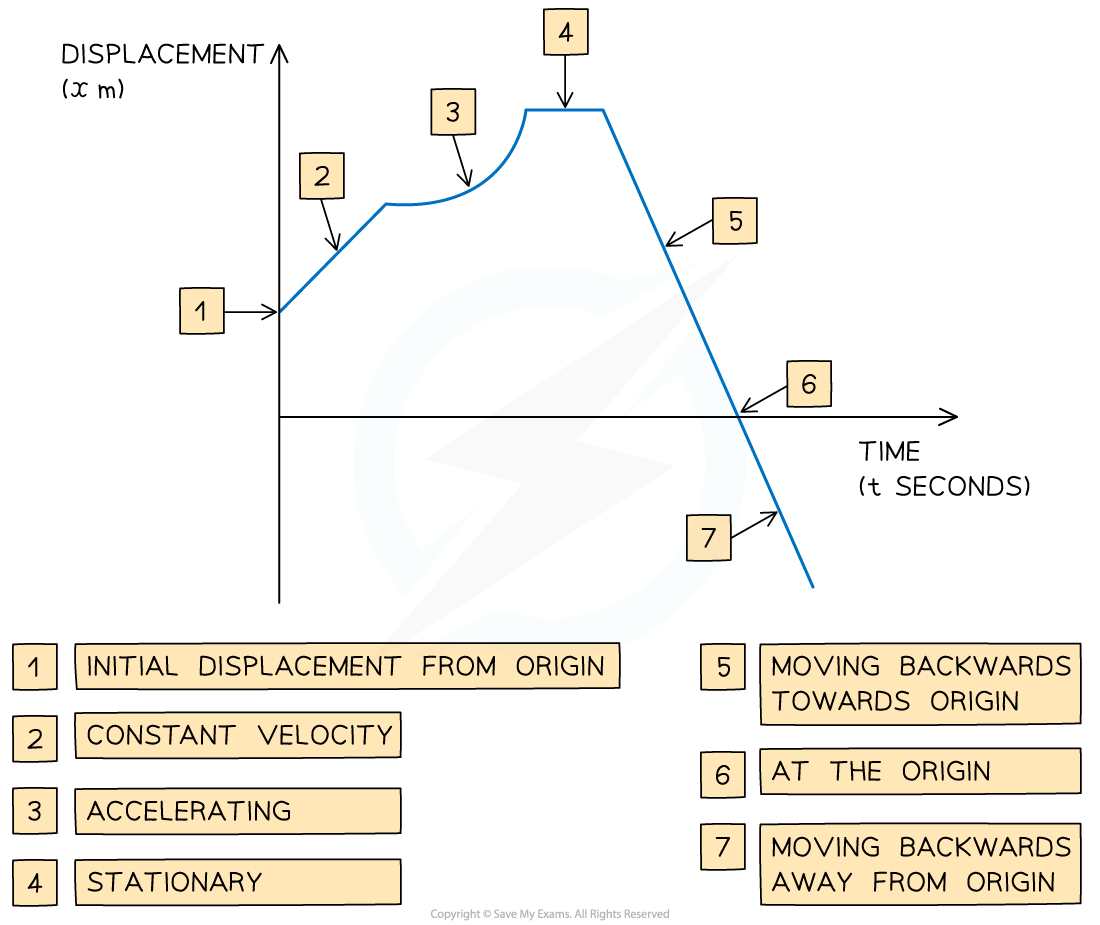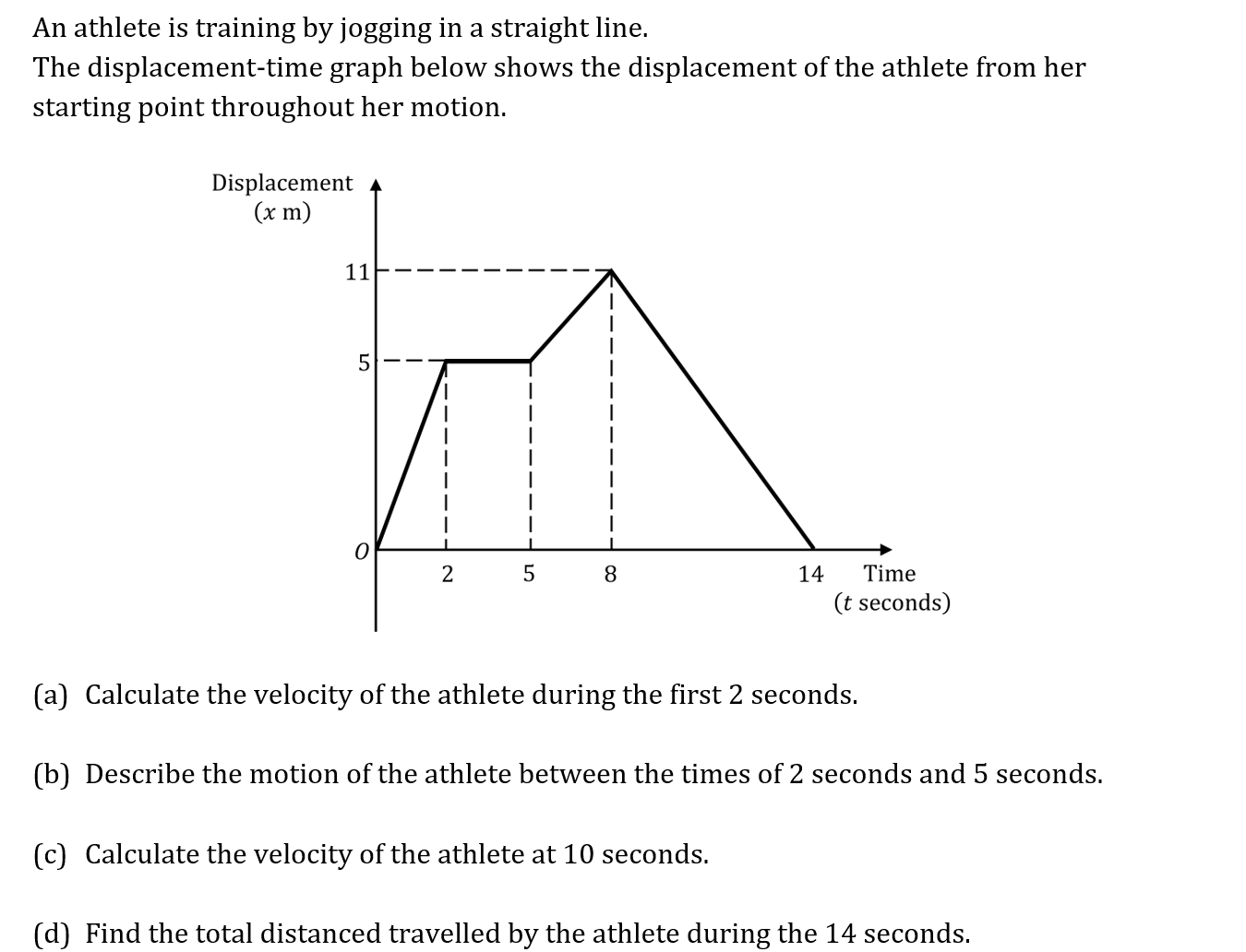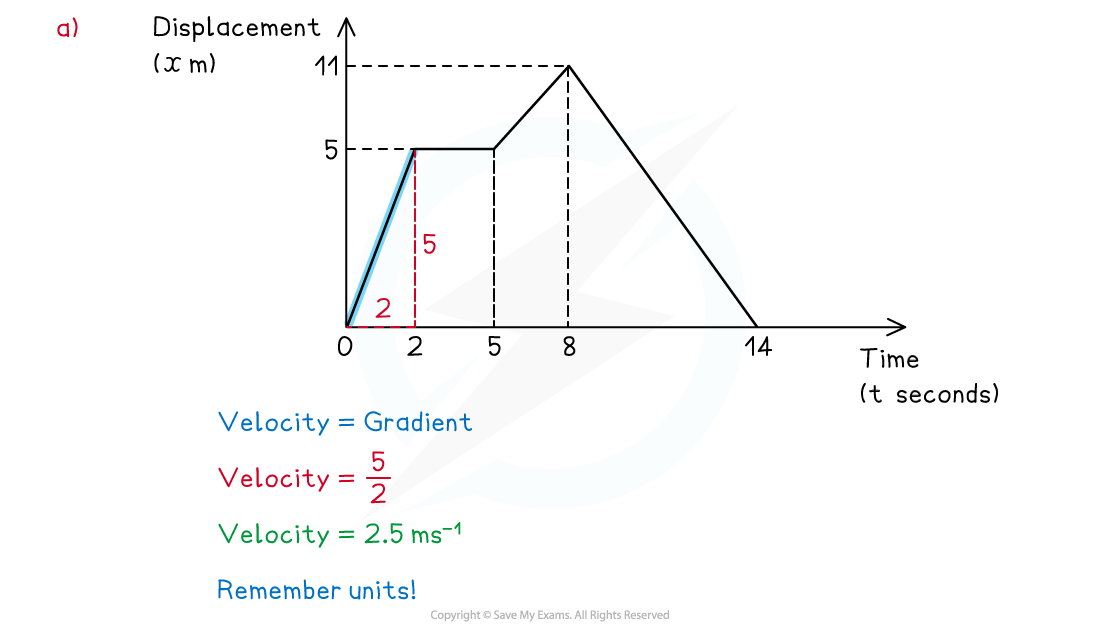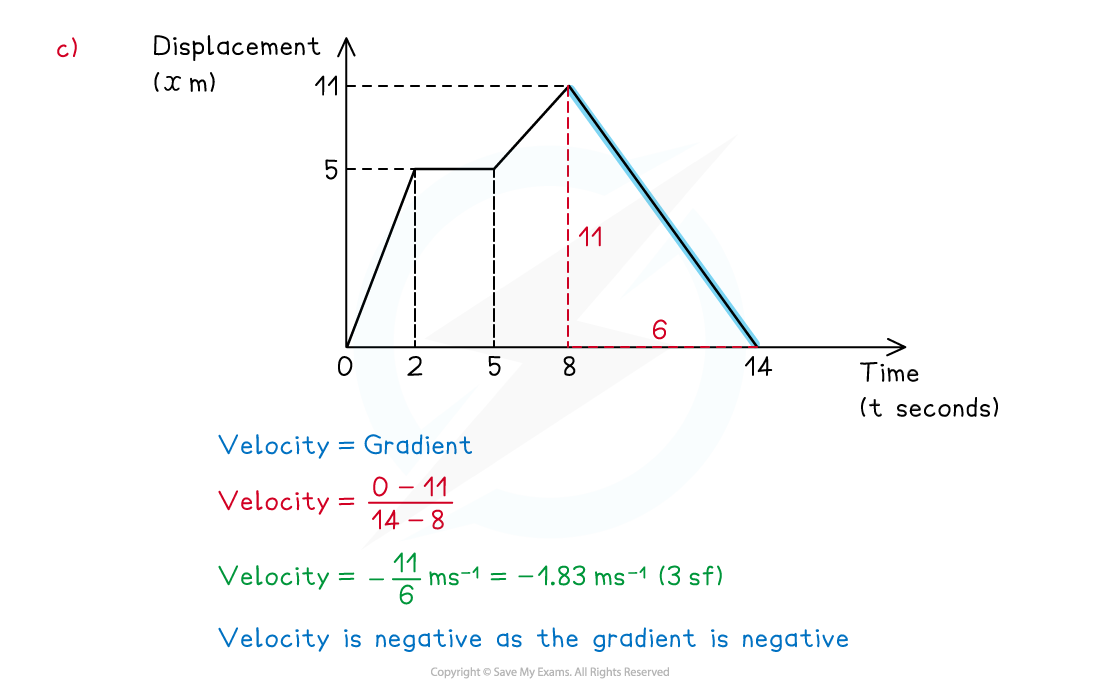# CIE A Level Maths: Mechanics复习笔记2.1.1 Displacement-Time Graphs

### Displacement-Time Graphs

#### What is a displacement-time graph?

• Displacement-time graphs show the displacement of an object from a fixed origin as it moves in a straight line
• They show displacement (on the vertical axis) against time (on the horizontal axis)
• Displacement-time graphs can go below the horizontal axis whereas distance-time graphs can not
• Distance can not be negative whereas displacement can be

#### What are the key features of a displacement-time graph?

• The gradient of the graph equals the velocity of the object
• positive gradient means the object is travelling forwards
• A negative gradient means that the object is travelling backwards
• The steeper the line, the greater the speed
• straight line shows that the object is moving at a constant velocity
• curved line shows that the object is accelerating or decelerating
• horizontal line shows that the object is stationary
• If the graph touches the x-axis, then the object is at the origin at that time#### Worked Example(a)   Calculate the velocity of the athlete during the first 2 seconds.(b)    Describe the motion of the athlete between the times of 2 seconds and 5 seconds.(c)   Calculate the velocity of the athlete at 10 seconds.(d)   Find the total distanced travelled by the athlete during the 14 seconds.#### Exam Tip

• Be careful to spot if you are working with a displacement-time graph or a velocity-time graph.
• Be careful to spot if you are working with a displacement-time graph or a distance-time graph
• Check where the graph starts from on the y-axis, the object does not have to start at 0. For example, if the graph starts at 100, the scenario could be a student on a walk starting 100 m from their house.
• Distance is a scalar so it can not be negative whereas displacement from a starting point can be.
• Think about the units when calculating, make sure they are consistent.